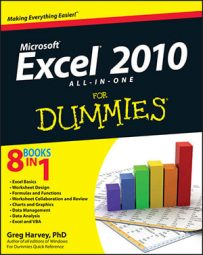##### Excel 2010 All-in-One For DummiesIf Excel 2010 can't properly calculate a formula that you enter in a cell, the program displays an error value in the cell as soon as you complete the formula entry. Excel uses several error values, all of which begin with the number sign (#).

## Excel's error values

The following table shows Excel's error values along with the meaning and the most probable cause for its appearance. To remove an error value from a cell, you must figure out what's wrong with the formula and fix it.

Error Values in Excel
Error Value Meaning Causes
#DIV/0 Division by zero The division operation in your formula refers to a cell that contains the value 0 or is blank.
#N/A No value available Technically, this is not an error value but a special value that you can manually enter into a cell to indicate that you don't yet have a necessary value.
#NAME? Excel doesn't recognize a name This error value appears when you incorrectly type the range name, refer to a deleted range name, or forget to put quotation marks around a text string in a formula.
#NULL! You specified an intersection of two cell ranges whose cells don't actually intersect Because a space indicates an intersection, this error will occur if you insert a space instead of a comma (the union operator) between ranges used in function arguments.
#NUM! Problem with a number in the formula This error can be caused by an invalid argument in an Excel function or a formula that produces a number too large or too small to be represented in the worksheet.
#REF! Invalid cell reference This error occurs when you delete a cell referred to in the formula or if you paste cells over the ones referred to in the formula.
#VALUE! Wrong type of argument in a function or wrong type of operator This error is most often the result of specifying a mathematical operation with one or more cells that contain text.

If a formula in your worksheet contains a reference to a cell that returns an error value, that formula returns that error value as well. This can cause error values to appear throughout the worksheet, thus making it very difficult for you to discover which cell contains the formula that caused the original error value so that you can fix the problem.

## Using the error alert button

When a formula yields an error value (other than #N/A) in a cell, Excel displays a green triangular error indicator in the upper-left corner of the cell and an alert options button appears to the left of that cell when you make it active.

If you position the mouse pointer on that options button, a ScreenTip appears, describing the nature of the error value. Also, a drop-down button appears to its right that you can click to display a drop-down menu with the following options:

• Help on This Error: Opens an Excel Help window with information on the type of error value in the active cell and how to correct it.

• Show Calculation Steps: Opens the Evaluate Formula dialog box, where you can walk through each step in the calculation to see the result of each computation.

• Ignore Error: Bypasses error checking for this cell and removes the error alert and Error options button from it.

• Edit in Formula Bar: Activates Edit mode and puts the insertion point at the end of the formula on the Formula bar.

• Error Checking Options: Opens the Formulas tab of the Excel Options dialog box, where you can modify the options used in checking the worksheet for formula errors.

If you're dealing with a worksheet that contains many error values, you can use the Error Checking button in the Formula Auditing group on the Ribbon's Formulas tab to locate each error.